# Artificial Intelligence - Part 2.2

## State-Space and Informed Search

Posted by Utkarsh Jain on May 18, 2020 · 22 mins read

This is the Part 2.2 in the Introduction to A.I. blog series. Checkout Part 1 and Part 2.1.

In the previous blog, I tried to introduce the concept of artificial intelligence, why it is needed and the different ways to achieve it. The main topics for this blog are:

## 1. Understanding Heuristic Functions (Lower-bound functions)

All of the search methods in the last blog are uninformed in the sense that they did not take into account the goal. They do not use any information about where they are trying to get to unless they happen to stumble on a goal. We saw how this can result in expanding unnecessary nodes and moving in a random direction. Is it possible to reduce this inefficiency by introducing some “vision” in the algorithm? What if we could give the search algorithm a sense of the direction of the goal state. This way we would make our search more informed and expand only those nodes which leads us to the goal.

One form of information about which nodes seem the most promising is a heuristic function h(n), which takes a node n and returns a non-negative real number that is an estimate of the path cost from node n to a goal node. A heuristic function should be informative in the sense that it helps choose those states that are likely to be on the optimal path or on short paths to the goal states. It is a function that helps to inform the search about the direction to a goal.

There is, however, a trade-off between informativeness and the cost of computing the heuristic function (h-function): computing the cost of the actual optimal solution is, of course, the same problem we are trying to solve, so using the cost of the shortest path to a goal state as the h-function does not make sense. In general, very expensive h-functions do not pay off: their ability to reduce the number of states visited is not sufficient when considering the per-state cost of computing them. Generally speaking, an h-function should be relatively cheap (low polynomial time) but still give useful information about the remaining cost.

A good example in the route planning or road navigation problem is the Euclidian (for 2-dimensional) which can be computed very quickly for any coordinates in the 2-dimensional plane as shown in the figure below.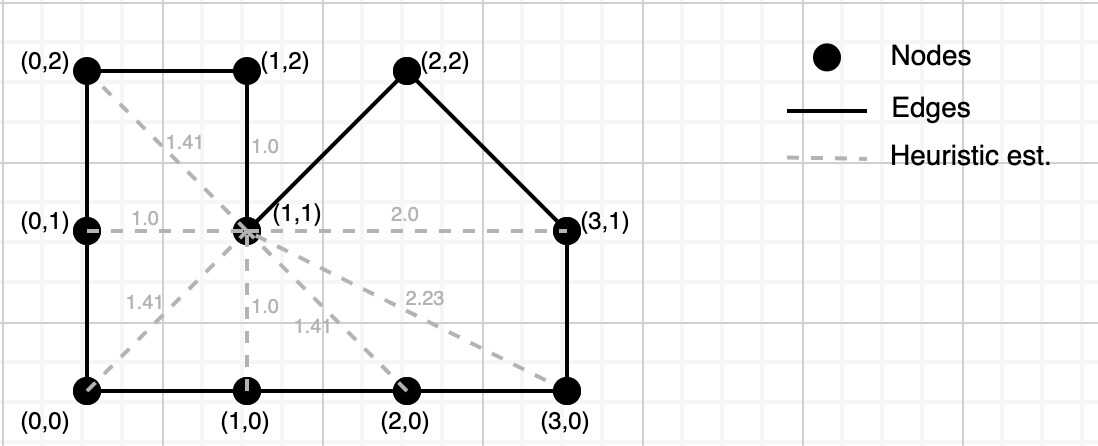## 2. Greedy Best-First Search

The greedy best-first search algorithm is obtained as a simple extension of the breadth-first search algorithm. Replace the FIFO queue by a priority queue, and associate each state with a value h(s) when inserting into the queue. Here h(s) is the heuristic value of reaching a goal state from a state s.

Pseudocode:

Inputs: a state-transition graph G = <N, A>, a heuristic function h: N → N, a start node s, and a goal node g.

Output: a solution path or failure

Insert h(s),s to the priority queue

While priority queue is not empty:

1. Remove a node n with the lowest priority h(n)
2. if n != g: Expand n and insert its successor into priority queue

If g was encountered during the search, follow the path from g to s using predecessors recursively, reverse it to a path from s to g, and return it.

Otherwise, return failure.

In the illustration below we can see how using heuristic function has benefitted us with lower search time as compared to the basic breadth-first search.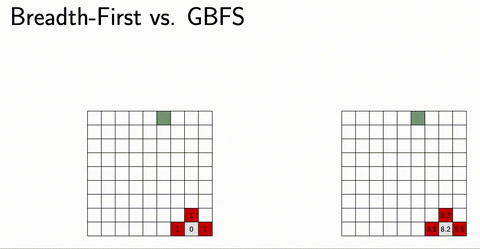The main problem in applying GBFS to complex problems is – in addition to actually formalizing that problem – devising effective heuristic functions. Not any function will do, and it may require lots of expertise in devising lower bound estimate functions that are effective in hard decision-making and planning problems. Problems that are not solvable with poor heuristic functions may suddenly become easily solvable with a better heuristic function.

## 3. A* Algorithm

We already saw the Greedy Best-First Search (GBFS) algorithm, which uses a search heuristic to guide the search in a promising direction. GBFS can be implemented as a simple modification of BFS, thereby not signifying a big departure from it. Although GBFS can reach goals much faster than BFS can, it can do it at the cost of a poor solution.

In this section, I will take the idea of heuristic functions more seriously, and consider their use in algorithms that find optimal solutions, that is, solutions that are guaranteed to be the best possible, in terms of the sum of the costs of the actions in them.

In this section, I will concentrate on the best known such algorithm, called A* (pronounced A-star). It can prune the search space substantially using h-functions for the costs of paths when deciding where the search proceeds next and simultaneously guaranteeing that only those states are considered that are necessary to either reach a goal state or to prove that the solution that has been found or is to be found. A* is, therefore, optimal also in that it never expands states unnecessarily (for a given heuristic function and given that we want to have a guarantee that the solutions are optimal.)

Definition: The A* algorithm approximates the cost of the path from the start node to the goal node and traversing through a node n using a heuristic function f(n) = g(n) + h(n) where

1. g(n) is the (known) path cost from the start node to n and,
2. h(n) approximates the remaining cost from n to the goal node.

Assumption: For all goal nodes n, h(n) = 0.

The key idea of the A* algorithm is to always expand a node n with the lowest estimated path cost f(n).In this way, A* can be seen as a combination of two-component search strategies:

• uniform-cost search which would expand a node n with lowest g(n) first, and
• greedy best-first search which would expand a node n with the lowest h(n) first.

I will now formulate the A* algorithm as close to the generic search algorithm as possible. However, I will make the use of the priority queue explicit in the formulation. The elements inserted to the queue are of the form <f(n),n> where f(n) is the priority value for the node n, which is the sum of the cost so far and the estimated (lower bound) remaining cost. For each node n, the algorithm keeps track of the known minimum distance min[n] from the start node as well as the previous node prev[n] on the path entering n.

Pseudocode:

Inputs: a state-transition graph G = <N, A>, a cost function c : A → N, a heuristic function h : N → N, a start node s, and a goal node g.

Outputs: a solution path and its length or failure.

For every node n, let min[n] := infinity and prev[n] = n.

Insert <h(s),s> to the priority queue and let min[s]=0.

While the priority queue is not empty:

1. Remove a node n with the highest priority (i.e., the least value of f(n)) from the queue.
2. If f(n) < min[g], then for each edge <n, m> in A such that min[n] + c(n,m) < min[m]:
3. Let min[m] := min[n] + c(n, m).
4. Let prev[m] := n.
5. If m != g: insert <min[m] + h(m), m> to the priority queue.

If g was encountered during the search, follow the path from g to s using predecessors recursively, reverse it to a path from s to g, and return it together with the distance min[g].

Otherwise, return failure.

The distance min[n] is initialized as infinity for each node n. Whenever min[n] < infinity holds, we know that n has been visited at least once, but it is highly possible that a particular node n is visited multiple times while f(n) is improved. The search starts from s which is inserted to the priority queue first, removed immediately, and then expanded. The expansion of a node n removed from the queue is guarded by the condition f(n) < min[g] which is void until the goal node g is reached for the first time because min[g] is initialized as infinity and f(n) is always finite. Once activated, this condition filters away nodes whose expansion cannot improve the value of min[g]. As regards the expansion of a particular node n, the immediate successors m of n in G are considered. Note that if m has not yet been visited min[m] = infinity, meaning that g(m) and f(m) are computed for the first time and m is inserted to the queue. However, there is no need to insert the goal node g to the queue at this point, because its heuristic score cannot be further improved through g itself. The value f(m) is obtained as min[m] + h(m) because g(n) = min[n] and g(m) = g(n) + c(n, m). In particular, if m = g, then min[g] coincides with f(g), i.e., the length of the current path entering g. When the algorithm terminates, this value coincides with the length of the optimal route to be reported by the algorithm.

Let us consider the actions of the robot in the order N, S, E, and W. The figure below illustrates how the search space is traversed by the A* algorithm as well as the path found from S to G in red color.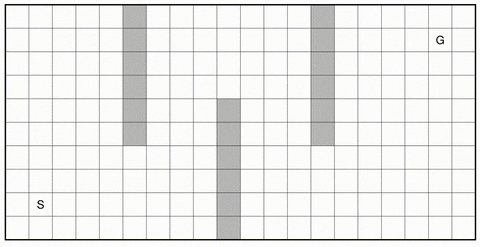### Analysis and Efficient Implementation

Since A* revisits nodes, the termination of the algorithm might become a concern. The essential observation is that a node n is revisited only if the value of f(n) is improved. Effectively this means that g(n) is improved since h(n) is constant and because a shorter route to n has been found. Therefore, the termination of A* essentially depends on two assumptions that are already incorporated in the formulation of A* above.

1. Once g(n), the value stored in min[n], is computed for the first time, it is a finite (natural) number. This means that g(n) can be improved only finitely many times. Thus we know that each node shall be revisited only finitely many times.
2. The state-transition graph G is explicitly enumerated and it is given as part of the input. Thus G is finite and there are only finitely many nodes and transitions to consider.

Under the assumptions above, the A* algorithm is bound to terminate. However, if the assumptions are defeated, termination could be endangered. In particular, if G is given implicitly, unbounded paths might result, even if we avoid loops by keeping track of visited nodes. The table min[n] serves this purpose and it can be implemented so that its size is not fixed when the execution of the algorithm starts, allowing the table to grow while the search proceeds. By the discussion above, the A* algorithm terminates for finite graphs, implying completeness. The optimality of A* depends on the properties of the heuristic function h(n).

### Example

All of the discussion until now was too theoretical. Let us now take up an example and see how both these algorithms work in practice. I will use the same graph I used in section 1 of this blog for explaining the algorithms.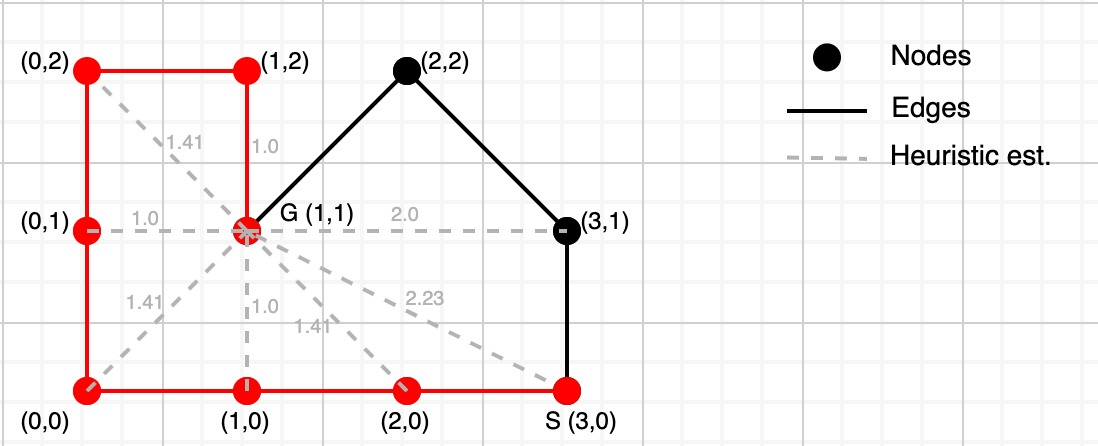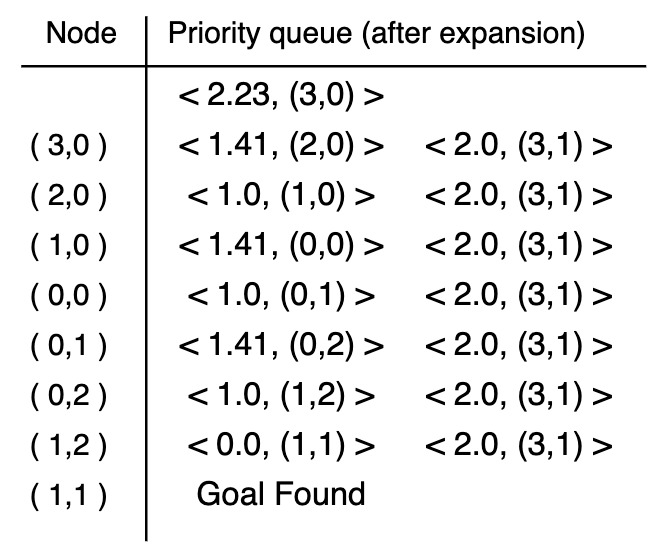Let us analyze the operation of the GBFS algorithm and priority queue resulting from expansions in more detail. The first step corresponds to the initialization of the algorithm from the source node S at coordinates (3,0). Then push the node along with its h-value of 2.23 in the priority queue. Next, we remove the node from the queue and expand it. The first expansion will result in pushing <(2,0), 1.41> and <(3,0), 2.0> into the queue. Next, we will remove the node with the lowest priority i.e (2,0), and expand it. As a result, node (1,0) is visited and we push it in the queue with its h-value of 1.0. We remove (1,0) from the queue because of the lowest priority and push its successor into the queue. In this way, the search proceeds until the goal node G at (1,1) is found. The solution path can be found by backtracking which is colored red in the image.

If we pay a little attention, we can clearly see that GBFS returned an inefficient solution. The optimal solution would have been to follow the path (3,0)→(3,1)→(2,2)→(1,1). Hence we see that GBFS doesn’t always return the optimal solution.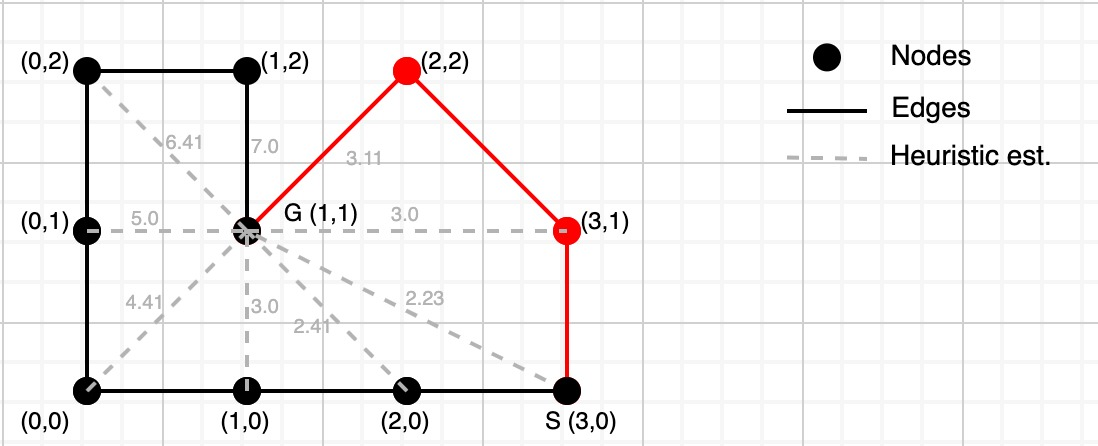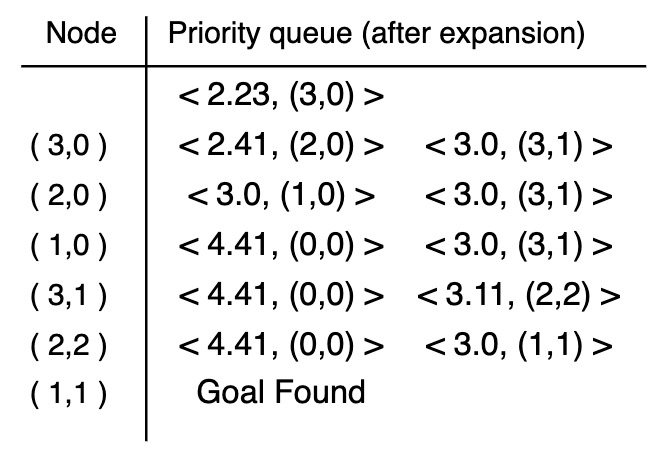Let us analyze the operation of the A* algorithm and priority queue resulting from expansions in more detail. The first step corresponds to the initialization of the algorithm from the source node S at coordinates (3,0). Then push the node along with its h-value of 2.23 in the priority queue. Next, we remove the node from the queue and expand it. The first expansion will result in pushing <(2,0), 2.41> and <(3,0), 3.0> into the queue. Next, we will remove the node with the lowest priority i.e (2,0), and expand it. As a result, node (1,0) is visited and we push it in the queue with its h-value of 3.0. Now we have two nodes with equal priority and one is selected arbitrarily. Let us select (1,0) which results in pushing <(0,0), 4.41> into the queue. Now we select (3,1) and expand it. In this way, the search proceeds until the goal node G at (1,1) is found. The solution path can be found by backtracking which is colored red in the image.

A* and GBFS aren’t the only algorithms we have to carry out an informed search. They both have their own pros and cons. A* guarantees optimality but has high runtime. Sometimes most of the work performed by A* takes place after finding the optimal solution. Greedy Best-First has low runtimes but doesn’t guarantee optimality. Some problems are too difficult to solve optimally in practice, and for some others having a little worse solution faster would be preferable. Is it possible to devise an algorithm that has a low runtime but can still provide a close-to-optimal solution? Yes, it is and WA* algorithm answers to these concerns. Unfortunately, WA* algorithm is not included in this blog but it is fairly simple to understand if you know how GBFS and A* works.

### Summing Up:

In this blog, we saw how we can use heuristic functions to make our search algorithm a bit more intelligent. We saw how their use can give a substantial gain in the performance and reduce the search time. We also noticed how GBFS, although runs faster than BFS, can end up giving a suboptimal path. To get a guaranteed optimal path we changed our heuristic function to include the previously known cost with the estimated cost.

Until this blog we looked at decision making in an environment will full observability and information. Next blog onwards I will talk about systems with partial observability and incomplete information. We will talk about Markov decision processes and how probability plays a big role in such processes and systems.

See you in the next blog!

### Source Code:

``````'''
Functions in classes representing state-space search problems:
successors: Returns [(a1,s1,c1),...,(aN,sN,cN)] where each si is
the successor state when action called ai is taken,
and ci is the associated cost.
Here the name ai of an action is a string.
'''
def ASTAR(initialstate,goaltest,h):
visited = dict() # dictionary for visited states
predecessor = dict() # dictionary for predecessors
g = dict() # dictionary for holding cost-so-far

q = queue.PriorityQueue()
q.put((h(initialstate), initialstate))
predecessor[initialstate] = None
g[initialstate] = 0
visited[initialstate] = 1
goalcost = float("inf")

while not q.empty():
x, current = q.get()
if(x < goalcost):
for a,state,cost in current.successors():
new_cost = g[current] + cost
if (state not in g.keys()):
g[state] = new_cost
predecessor[state] = current
priority = new_cost + h(state)
q.put((priority,state))
if(goaltest(state)):
goalcost = new_cost
return (predecessor, goalcost)

'''
ASTAR returns a pair (plan, cost) where
plan is the sequence of states on an
optimal path to goals, cost is the sum
of the costs of actions on that path.
'''
``````

Checkout Part 1 for basic introduction to A.I. and Part 2.1 to learn about uninformed search methods in A.I.

References:

• S. Russel and P. Norvig: Artificial Intelligence: A Modern Approach, Chapters 3 and 4, 3rd Edition, 2010.

Note: If you think there’s anything wrong in this article or something that can be improved in any way, feel free to point out in the comments.